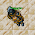## Wednesday, June 16, 2021

### TibiaGoals Riddles E9R06

Do you know the answer?
Comment down below!

1.2.Nehkz
Relania

The answer is 34.

3.Nehkz
Relania

The answer is 34.

4.Nehkz
Relania

The answer is 34.

5.Hoi! My answer is 28. :) Thanks!

6.The answer is 28 because crown is 7, soft boots is 12 (but the final equation is half of the pair so 6), and golden helmet is 15 so

7.7+15+6=28

Xarkost Eilia

8.9.Golden Helmet = 15
Soft Boots = 12 (One Soft boot = 6)
Crown = 7

7 + 15 + 6 = 28

Char: Dyaniixz

10.The answer is 28!

Pinguzz

11.7+15+6=28
Ray Jek

12.28
Mian Stone'arrow

13.14.28

Skyelie Hasulhoff <3

15.16.Neo

Golden helmet = 15
Pair of soft boots = 12
Crown = 7
Single soft boot = 6
7+15+6=28

17.15+15+15=45
15+12+12=39
12-7=5
7+15+6=28..... Bebitha consentida

18.15+15+15=45
15+12+12=39
12-7=5
7+15+6=28..... Bebitha consentida

19.20.21.7+15+6= 28
By Aurora Ventresca

22.23.Nehkz
Relania

I have another answer, the previous was wrong :D It is:
7+15+6=28

24.Nehkz
Relania

I have another answer, the previous was wrong :D It is:
7+15+6=28

25.Nehkz
Relania

I have another answer, the previous was wrong :D It is:
7+15+6=28

26.28
Shizurie

27.28 Lord Neganek

28.28 shizurie

29.30.The answer is: 28

Char name: Fyori

31.character name: pablo reformed

32.28 /Norelli

33.28
Character: Amy Meow

34.15 + 15 + 15 =45
15 + 12 + 12 =39
12 - 7 = 5
7 + 15 + 6 = 28

Toxic Hasulhoff

35.31

pameliin

36.Golden helmet= 15
Soft boots= 12
Crown= 7
15+12+7=34
Eternal hunger
Antica

37.Golden helmet= 15
Soft boots= 12
Crown= 7
15+12+7=34
Eternal hunger
Antica

38.Golden helmet= 15
Soft boots= 12
Crown= 7
15+12+7=34
Eternal hunger
Antica

39.28

Lovely olka

40.R=28
samuray de carro :)

41.28 Demonovsky

42.Congratulations! Correct answer is: 28.

See you on Sunday!

If you have any questions or doubts, don't hesitate to contact us at Tibiagoals.adm@gmail.com